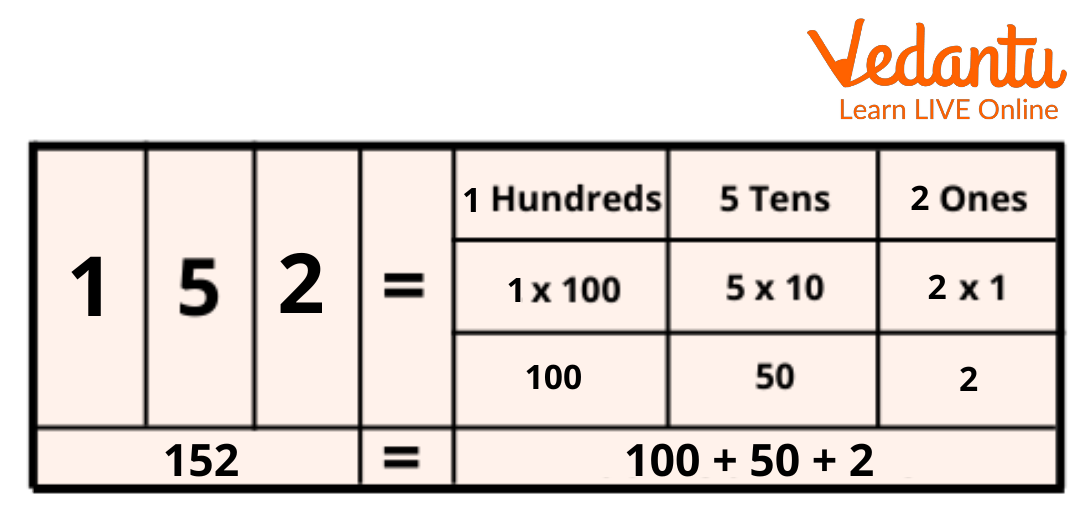Courses
Courses for Kids
Free study material
Free LIVE classes
More

# The Expanded Form of Decimals and Place Value System## What is the Expanded Form?

The expanded form of decimals is a notation for the decimal numbers, which is the mathematical expression to represent the sum of the values of each digit in the number. We use the place value system to write the expanded form of any number. In mathematics, the value of each digit can be written in expanded form by showing the number as a sum of each digit multiplied by its place value.

In this article, we will learn about the place value system which will help us to understand the expanded form of a decimal.

## Place Value System

The place value in the place value system refers to the value of a digit in a number. The value for each number is calculated using the position of the number. Starting from right to left, we can understand the notations used in the place value with the help of an example. The place value chart, which is used in the expanded form, is given below.Place Value Chart

Let’s take the number 152. For this number, the place value chart is drawn below.Place Value Chart of 152

Hence, the expanded form of the number 152 is 100 + 50 + 2.

In the same way, when we want to write the expanded form of a decimal or fractional number, it is written with a base 10-multiple denominator which is represented by the power of 10. For example, we can take the decimal number 3.482. In the expanded form, it is written as follows.The Place Value Chart of 3.482

Hence, the expanded form of the decimal number 3.482 is as follows:

= 3 + $\frac{4}{10} + \frac{8}{100} + \frac{42}{1000}$

= 3 + 0.4 + 0.08 + 0.002

## Uses of the Expanded Form of Decimals

• The scope of the expanded forms of decimals lies in some areas where accuracy and precision are needed.

• The concept of the expanded form is helpful in comprehending the value of a number and the numeric value of a quantity.

## Do You Know?

• Mathematician Archimedes was the inventor of a decimal positional system for Sand Reckoner to show large numbers which were multiples of 10.

• For trade purposes, weights in the ratios of $\frac{1}{20} , \frac{1}{10} , \frac{1}{5} , \frac{1}{2}$ and multiples of 10 were used in the Indus Valley Civilization.

• In ancient China, Rod calculus used bamboo strips with the decimal system for mathematical operations like multiplication during 305 BC.

## Conclusion

The expanded form of a number helps us to understand the number better. In this article, the expanded form of decimals is explained through examples. It is difficult to understand a large number but when it is written in the expanded form, we can understand it easily because of its place value.

Last updated date: 28th May 2023
Total views: 93k
Views today: 0.60k

## FAQs on The Expanded Form of Decimals and Place Value System

1. What is the expanded form of decimals?

The decimal numbers can be represented in the expanded form. We multiply each of the decimal digits with increasing exponent values of (1/10) to write a number in the expanded form. We can understand this with the help of an example of a decimal number.

The decimal number 0.347 can be written in the expanded form as 3 × (1/10) + 4 × (1/10)2 + 7 × (1/10)3

= 3 × (1/10) + 4 × (1/100) + 7 × (1/1000)

= 0.3 + 0.04 + 0.007

2. What will 33.24 be when written in the expanded form?

We know that the digits after the decimal point are represented as tenth (1/10), hundredth (1/100), thousandth (1/1000) and so on. Now, let us consider the example of 33.24. The expanded form of 33 is 30+3. The expanded form of the number can also be represented as 30+3+0.2+0.04.